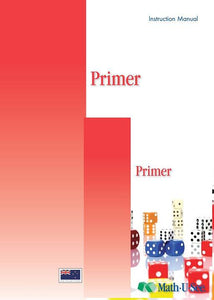or 6 weekly interest-free payments from \$14.16 withwhat's this?Demme Learning

# Math.U.See Primer

Regular price \$49.00 \$46.07 Unit price per
Tax included. Shipping calculated at checkout.

## Math.U.See Primer

##### an introduction to Mathematics

In the Primer level your child will learn not only how to write numerals but also addition and subtraction, basic counting, skip counting, geometric shapes, telling time, and they will be introduced to the manipulative block system. It’s an introduction to "doing math."

Major Concepts and Skills Include:

• Counting objects and developing numeracy
• Understanding place value
• Recognizing number names and symbols
• Understanding addition and subtraction with concrete and representational models
• Understanding and writing the symbols +, – and =
• Addition and subtraction of select numbers

• Telling and writing time by hours and minutes
• Recognizing & drawing rectangles, squares, and circles
• Measuring length by repeating units
• Introducing halves and fourths
• Counting by 2s, 5s, 10s, and 100s
• Reading, writing & interpreting word problem

Primer Instruction Pack includes: Instruction Manual & DVD

Primer Student Workbook includes: Student workbook - grab an extra workbook for an additional Student.

For a complete set to get started with Math.U.See you will need to purchase the Integer Black set (a one of purchase for use with ALL levels of Math.U.See), the Primer instruction pack & Primer Student Workbook.